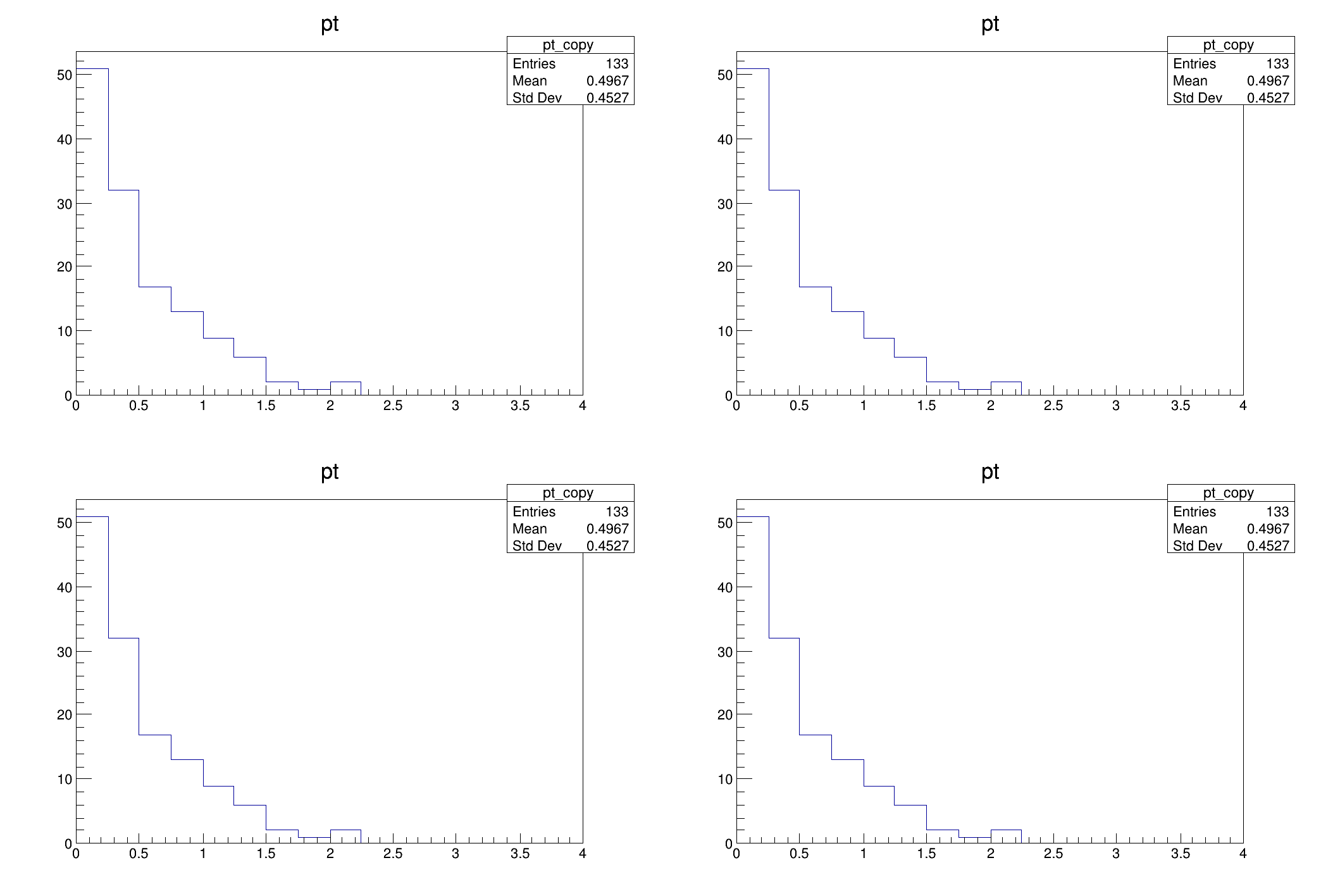ROOT   Reference Guide
Searching...
No Matches
df017_vecOpsHEP.C File Reference

## Detailed DescriptionUse RVecs to plot the transverse momentum of selected particles.

This tutorial shows how VecOps can be used to slim down the programming model typically adopted in HEP for analysis. In this case we have a dataset containing the kinematic properties of particles stored in individual arrays. We want to plot the transverse momentum of these particles if the energy is greater than 100.

auto filename = gROOT->GetTutorialDir() + "/dataframe/df017_vecOpsHEP.root";
auto treename = "myDataset";
using RDF = ROOT::RDataFrame;
{
TFile f(filename);
TH1F h("pt", "pt", 16, 0, 4);
while (tr.Next()) {
for (auto i=0U;i < px.GetSize(); ++i) {
if (E[i] > 100) h.Fill(sqrt(px[i]*px[i] + py[i]*py[i]));
}
}
h.DrawCopy();
}
void WithRDataFrame()
{
RDF f(treename, filename.Data());
auto CalcPt = [](doubles &px, doubles &py, doubles &E) {
doubles v;
for (auto i=0U;i < px.size(); ++i) {
if (E[i] > 100) {
v.emplace_back(sqrt(px[i]*px[i] + py[i]*py[i]));
}
}
return v;
};
f.Define("pt", CalcPt, {"px", "py", "E"})
.Histo1D<doubles>({"pt", "pt", 16, 0, 4}, "pt")->DrawCopy();
}
void WithRDataFrameVecOps()
{
RDF f(treename, filename.Data());
auto CalcPt = [](doubles &px, doubles &py, doubles &E) {
auto pt = sqrt(px*px + py*py);
return pt[E>100];
};
f.Define("good_pt", CalcPt, {"px", "py", "E"})
.Histo1D<doubles>({"pt", "pt", 16, 0, 4}, "good_pt")->DrawCopy();
}
void WithRDataFrameVecOpsJit()
{
RDF f(treename, filename.Data());
f.Define("good_pt", "sqrt(px*px + py*py)[E>100]")
.Histo1D({"pt", "pt", 16, 0, 4}, "good_pt")->DrawCopy();
}
{
// We plot four times the same quantity, the key is to look into the implementation
// of the functions above
auto c = new TCanvas();
c->Divide(2,2);
c->cd(1);
c->cd(2);
WithRDataFrame();
c->cd(3);
WithRDataFrameVecOps();
c->cd(4);
WithRDataFrameVecOpsJit();
}
#define f(i)
Definition RSha256.hxx:104
#define c(i)
Definition RSha256.hxx:101
#define h(i)
Definition RSha256.hxx:106
double sqrt(double)
#define gROOT
Definition TROOT.h:406
ROOT's RDataFrame offers a high level interface for analyses of data stored in TTrees,...
A "std::vector"-like collection of values implementing handy operation to analyse them.
Definition RVec.hxx:296
The Canvas class.
Definition TCanvas.h:23
A ROOT file is a suite of consecutive data records (TKey instances) with a well defined format.
Definition TFile.h:54
1-D histogram with a float per channel (see TH1 documentation)}
Definition TH1.h:575
An interface for reading collections stored in ROOT columnar datasets.
A simple, robust and fast interface to read values from ROOT columnar datasets such as TTree,...